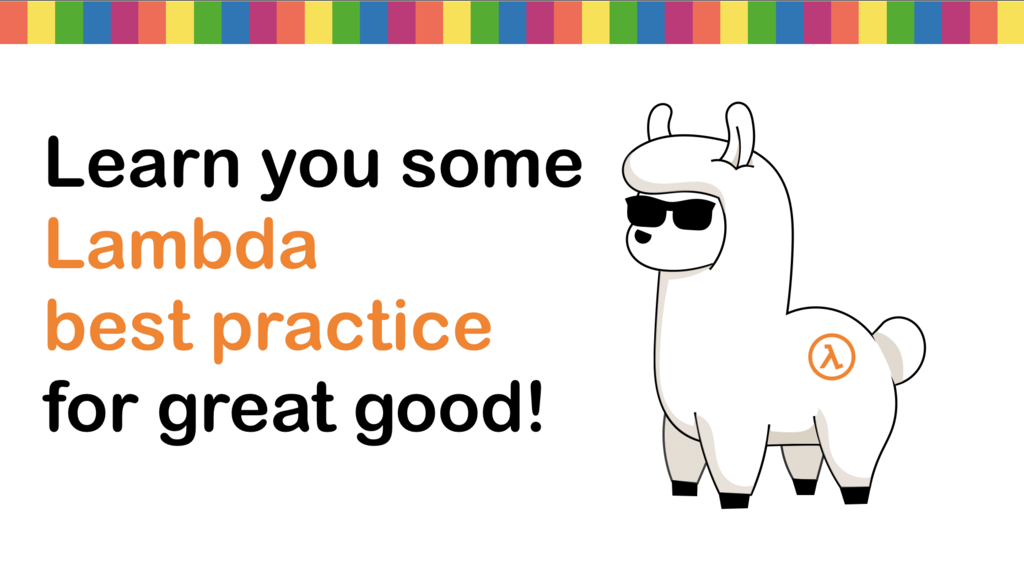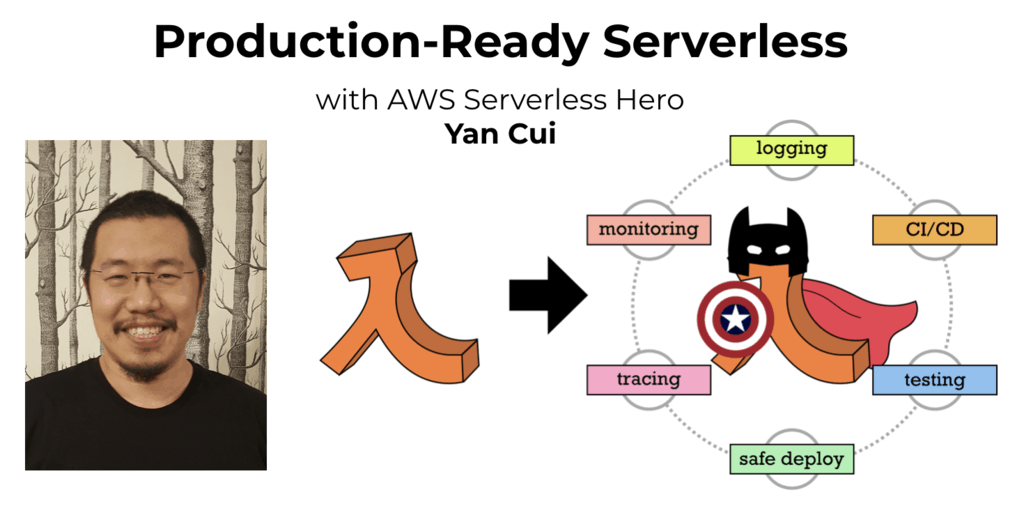# Project Euler – Problem 12 Solution

Check out my new course Learn you some Lambda best practice for great good! and learn the best practices for performance, cost, security, resilience, observability and scalability.

#### Problem

The sequence of triangle numbers is generated by adding the natural numbers. So the 7th triangle number would be 1 + 2 + 3 + 4 + 5 + 6 + 7 = 28. The first ten terms would be:

1, 3, 6, 10, 15, 21, 28, 36, 45, 55, …

Let us list the factors of the first seven triangle numbers:

1: 1

3: 1,3

6: 1,2,3,6

10: 1,2,5,10

15: 1,3,5,15

21: 1,3,7,21

28: 1,2,4,7,14,28

We can see that 28 is the first triangle number to have over five divisors.

What is the value of the first triangle number to have over five hundred divisors?

#### Solution

```open System

let triangleNumber(n:int64) = [1L..n] |> Seq.sum

let findFactorsOf(n:int64) =
let upperBound = int64(Math.Sqrt(double(n)))
[1L..upperBound]
|> Seq.filter (fun x -> n % x = 0L)
|> Seq.collect (fun x -> [x; n/x])

let naturalNumbers = Seq.unfold (fun x -> Some(x, x+1L)) 1L

naturalNumbers
|> Seq.map (fun x -> triangleNumber(x))
|> Seq.filter (fun x -> Seq.length(findFactorsOf(x)) >= 500)
```

My solution here is simple, first create the triangleNumber function that calculates the triangle number for a given natural number.

I then created a modified version of the findFactorsOf function I first used in the problem 3 solution to find ALL the factors of a number including 1 and itself.

Again I used Seq.unfold to build up a sequence of natural numbers and to find the answer I iterate through the natural numbers and for each get its triangle number; find the factors of the triangle number; and check if the number of factors is at least 500.Hi, I’m Yan. I’m an AWS Serverless Hero and the author of Production-Ready Serverless.

I specialise in rapidly transitioning teams to serverless and building production-ready services on AWS.

Are you struggling with serverless or need guidance on best practices? Do you want someone to review your architecture and help you avoid costly mistakes down the line? Whatever the case, I’m here to help.Check out my new course, Learn you some Lambda best practice for great good! In this course, you will learn best practices for working with AWS Lambda in terms of performance, cost, security, scalability, resilience and observability. Enrol now and enjoy a special preorder price of £9.99 (~\$13).Are you working with Serverless and looking for expert training to level-up your skills? Or are you looking for a solid foundation to start from? Look no further, register for my Production-Ready Serverless workshop to learn how to build production-grade Serverless applications!